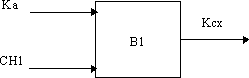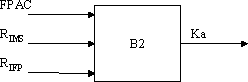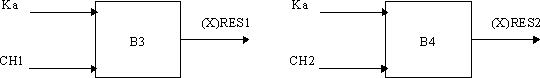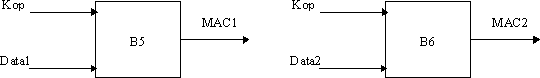## E.9 External specification of security related algorithms

3GPP43.020Release 16Security related network functionsTS

This annex specifies the cryptological algorithms and algorithms which are needed to provide the various security features and mechanisms defined in the CTS service description.

The following algorithms are considered;

– Algorithm A5/2: Ciphering/deciphering algorithm;

– Algorithm B1: Ciphering key generation algorithm

– Algorithm B2: Authentication key generation algorithm

– Algorithm B3: Authentication algorithm

– Algorithm B4: Authentication algorithm

– Algorithm B5: Message authentication algorithm used for CTS-FP authentication

– Algorithm B6: Message authentication algorithm used for signature authentication

The A5/2 is specified in 3GPP TS 43.020 Annex C.

The external specification of the algorithms B1, B2, B3, B4, B5, B6 is defined below. The internal specification is managed by SAGE.

## E.9.1 Algorithm B1

### E.9.1.1 Purpose

The B1 algorithm is used to generate the ciphering key Kc from the two random challenges CH1 and the authentication key Ka which is derived from Ka.

Location: CTS-ME, CTS-FPE

### E.9.1.2 Implementation and operational requirements

The two input parameters Ka, CH1 and the output parameter Kc of the algorithm shall use the following formats:Figure E13: The ciphering key generator B1

– Input 1: Bit string of length |Ka| = 128 bits;

– Input 2: Bit string of length |CH1| = 128 bits;

– Output: Bit string of length |Kc| = 64 bits.

The calculation time of B1 shall not exceed 200 ms.

## E.9.2 Algorithm B2

### E.9.2.1 Purpose

The algorithm B2 is used to generate:

– The authentication key Ka;

– The initial authentication key. This authentication key generation and usage is part of the initialisation method using the CTS Radio Interface.

Location: CTS-ME, CTS-FPE

### E.9.2.2 Implementation and operational requirements

The three input parameters FPAC , RIMS , RIFP, and the output parameterKa of the algorithm shall use the following formats:Figure E14: The key generation algorithm B2

– Input 1: Bit string of length |FPAC| respective bit string of length |FPAC| = 128 bit;

– Input 2: Bit string of length |RIMS| respective bit string of length |RIMS| = 64 bit;

– Input 3: Bit string of length |RIFP| respective bit string of length |RIFP| = 64 bit;

– Output: Bit string of length |Ka| = 128 bit.

The calculation time of the B2 algorithm shall not exceed 250 ms.

## E.9.3 Algorithms B3 and B4

### E.9.3.1 Purpose

The B3 and B4 algorithms are used to perform the mutual authentication via a challenge-response scheme.

Location: CTS-ME, CTS-FPE.

### E.9.3.2 Implementation and operational requirements

The two input parameters Ka and CH1 respective CH2 and the output parameter (X)RES1 respective (X)RES2 of the algorithm shall use the following formats:Figure E15: The response generation by B3 and B4

– Input 1: Bit string of length |Ka| = 128 bit;

– Input 2: Bit string of length |CH1| respective bit string of length |CH2| = 128 bit;

– Output: Bit string of length |(X)RESP1| respective bit string of length |(X)RESP2| = 128 bit.

The calculation time of B3 respective B4 shall not exceed 200ms for one operation.

## E.9.4 Algorithms B5 and B6

### E.9.4.1 Purpose

The B5 algorithm is used to perform CTS-FP authentication by the CTS-SN.

The B6 algorithm is used by the CTS-FP to authenticate the signature issued by the CTS-SN.

Location: CTS-FPE, CTS-SN.

### E.9.4.2 Implementation and operational requirements

The two input parameters Kop and Data1 respective Data2 and the output parameter MAC1 respective MAC2 of the algorithm shall use the following formats:Figure E16: The response generation by B5 and B6

– Input 1: Bit string of length |Kop| = 128 bit;

– Input 2: Bit string of length |Data1| respective bit string of length |Data2| = n octets;

– Output: Bit string of length |MAC1| respective bit string of length |MAC2| = 64 bit.